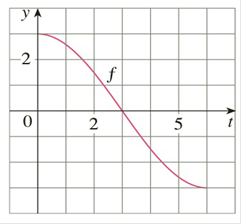Chapter 4.3, Problem 4E

Chapter
Section
Textbook Problem

# Let g ( x ) = ∫ 0 x f ( t ) d t where f is the function whose graph is shown.(a) Evaluate g ( 0 ) and g ( 6 ) .(b) Estimate g ( x ) for x = 1 , 2 , 3 , 4 ,  and 5 .(c) On what interval is g increasing?(d) Where does g have a maximum value?(e) Sketch a rough graph of g.(f) Use the graph in part (e) to sketch the graph of g ′ ( x ) . Compare with the graph of f.To determine

a)

To evaluate:

i. g0

ii. g6

Explanation

Given:

gx=0xf(t)dt

Calculation:

i. To evaluate g0

The integral of a function over a trivial interval is zero  so

g0=00f(t)dt=0

ii. To evaluate g6

The graph of f has rotational symmetry about the point (3, 0)

So the part from x=0 to 3 is equal in area to the part from x=3

To determine

b)

To estimate:

gx for x=1, 2, 3, 4 and 5

To determine

c)

To find:

On what interval is g increasing

To determine

d)

To find:

Where does g have the maximum value

To determine

e)

To sketch:

A rough graph of g

To determine

f)

To sketch:

A rough graph of g' and compare it to  f

### Still sussing out bartleby?

Check out a sample textbook solution.

See a sample solution

#### The Solution to Your Study Problems

Bartleby provides explanations to thousands of textbook problems written by our experts, many with advanced degrees!

Get Started

#### In Exercises 516, evaluate the given quantity. log100,000

Finite Mathematics and Applied Calculus (MindTap Course List)

#### (rn)4r52n

Applied Calculus for the Managerial, Life, and Social Sciences: A Brief Approach

#### Find the derivatives of the functions in Problems 1-34. 18.

Mathematical Applications for the Management, Life, and Social Sciences

Elementary Technical Mathematics

#### Sometimes, Always, or Never: The sum of two elementary functions is an elementary function.

Study Guide for Stewart's Single Variable Calculus: Early Transcendentals, 8th

#### True or False: is a convergent series.

Study Guide for Stewart's Multivariable Calculus, 8th

#### Explain the difference between passive and active deception.

Research Methods for the Behavioral Sciences (MindTap Course List)